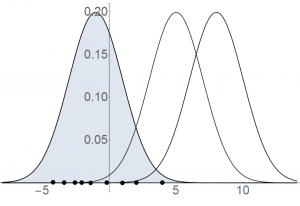23
Mar 17

## Maximum likelihood: idea and life of a bulb

Maximum likelihood: idea of the method and application to life of a bulb. Sometimes I plagiarize from my book.

### Maximum likelihood ideaFigure 1. Maximum likelihood idea

The main idea of the maximum likelihood (ML) method is illustrated in Figure 1. We start with the sample depicted with points on the horizontal axis. Then we think which of the densities shown on the figure is more likely to have generated that sample. Of course, it's the one on the left, filled with grey.

This density takes higher values at observed points than the other two. Note also that the position of the density is regulated by its parameters. This explains the main idea: choose the parameters so as to maximize the density at the observed points.

### Algorithm

Step 1. A statistical model usually contains a random term. To describe that term, choose a density from some parametric family. Denote it$f(x|\theta)$ where$\theta$ is a parameter or a set of parameters.$f(x_i|\theta)$ is the value of the density at the$i$th observation.

Step 2. Assume that observations are independent. Then the joint density is a product of own densities:$f(x_1,...,x_n|\theta)=f(x_1|\theta)...f(x_n|\theta)$. Since the observations are fixed, the joint density is a function of just parameters.

Definition. The joint density as a function of just parameters is called a likelihood function and denoted$L(\theta|x_1,...,x_n)=f(x_1,...,x_n|\theta)$, to reflect the fact that the parameters are the main argument. The parameters that maximize the likelihood function, if they exist, are the maximum likelihood estimators, and thus the name Maximum Likelihood (ML) method.

Step 3. Since$\log x$ is a monotone function, the likelihood$L(\theta |x_1,...,x_n)$ and the log-likelihood function$\lambda(\theta)=\log L(\theta|x_1,...,x_n)$ are maximized at the same time. The likelihood is often a multiplicative function, in which case maximizing the log-likelihood is technically easier.

Comments. (1) Most of the time the likelihood function is difficult to maximize analytically. Then maximization is done on the computer. A numerical algorithm can give the solution only approximately. Moreover, the likelihood function may not have maximums at all or may have many maximums; in the former case the numerical procedure does not converge and in the latter the computer gives only one solution.

(2) One should distinguish models and estimation methods. The OLS method applied to the linear model gives OLS estimators. The ML method applied to the same linear model gives ML estimators. Most linear models are dealt with using the least squares method. All exercises for maximum likelihood require some algebra, as is seen from the algorithm.

### Example: life of a bulb

Life of a bulb is described by the exponential distribution$p(t)=0$, if$t\le0$, and$p(t)=\mu{e^{-\mu t}}$ if$t>0$

where$\mu$ is a positive parameter. Life of a bulb cannot be negative, so the density is zero on the left half-axis. The density takes high values in the right neighborhood of the origin and quickly declines afterwards. That means that the probability that the bulb will burn right after it's produced is the highest, but if it survives the first minutes (hours, days), it will serve for a while. Most electronic products behave like this.

Exercise. Derive the ML estimator of$\mu$.

Solution. Step 1.$f(x_i|\mu)=\mu e^{-\mu x_i}$.

Step 2. Assuming independent observations, the joint density is a product of these densities$\mu^{n}e^{-\mu x_1}...e^{-\mu x_n}$.

Step 3. The log-likelihood function is$\lambda=n\log\mu-\mu(x_1+...+x_n)$. The first order condition is$\frac{\partial\lambda}{\partial\mu}=\frac{n}{\mu}-(x_1+...+x_n)=0$ and its solution is$\mu=\frac{1}{\bar{x}}$. To make sure that this is a maximum, we need to check the second order condition. Since$\frac{\partial^{2}\lambda }{\partial\mu^{2}}=-\frac{n}{\mu^2}$ is negative, we have really found the maximum.

Conclusion:$\hat{\mu}_{ML}=\frac{1}{\bar{x}}$ is the ML estimator for$\mu$.